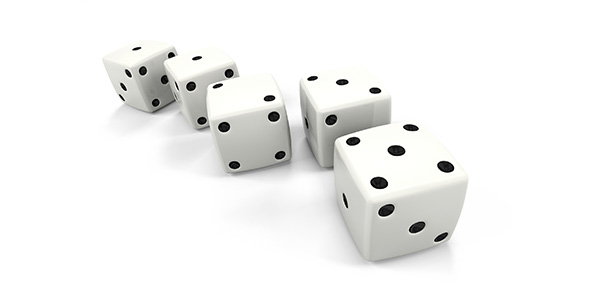# A Unique Quiz On Chapter 12: Probability

22 Questions | Total Attempts: 49SettingsThat’s right! When you roll a handful of dice or go all in on one number at the roulette table, there’s a lot more going on than just luck and random outcomes. Every game at a casino and many more aspects of life are directly affected by the mathematical topic of probability. What do you know about it?

• 1.
a controlled operation that yield a set of results.
• A.

Expected Value

• B.

Fair price

• C.

Experiment

• D.

Relative frequency

• 2.
the possible results of an experiment
• A.

Net Amount

• B.

Event

• C.

Mutually exclusive

• D.

Outcomes

• 3.
a subcollection of the outcomes of an experiment
• A.

Event

• B.

Law of large numbers

• C.

Expected Value

• D.

Sample space

• 4.
the relative frequency of occurrence of an event. It's determind by actual observation of an experiment. P(E) = number of times event E has occured/ number of times experiment was performed
• A.

Empirical probability (Relative frequency)

• B.

Equally likely outcomes

• C.

Compound probability

• D.

Fair price

• 5.
A kind of probabiltiy that is determined through a study of the possible outcomes that can occur for the given experiment.
• A.

Compound probability

• B.

Conditional probability

• C.

Theoretical probability

• D.

Empirical probability

• 6.
It states that probability statements apply in practice to a large number of trial, not to a single trial. It is the relative frequency over the long run that is accurately predictable, not individual events or precise totals.
• A.

Empirical probability

• B.

Law of large numbers

• C.

Equally likely outcomes

• D.

Compound probability

• 7.
If each outcomes of an experiment has the same chance of occurring as any other outcome
• A.

Mutually exclusive

• B.

Equally likely outcomes

• C.

Empirical probability

• D.

Expectation

• 8.
a ratio of the probability that the event will fail to occur (failure) to the probability that the event will occur ( success).
• A.

Net Amount

• B.

Expected Value

• C.

Odds in favor

• D.

Odds against

• 9.
a ratio of the probability that the event will occcur to the probability that the event will fair to occur.
• A.

Net Amount

• B.

Expected Value

• C.

Odds in favor

• D.

Odds against

• 10.
is often used to determine the expected results of an experiment or business venture over the long term. It's also used to predict the expected gain or loss in games of chance such as the lottery, roulette, craps, and slot machines.
• A.

Experiment

• B.

Expected Value (Expectation)

• C.

Fair price

• D.

Independent events

• 11.
It is a term commonly used in business accounting, although it is sometimes borrowed by personal finance professionals. It is often used interchangeably with net income. The personal finance definition of it refers to the income left over after taxes have been deducted, often called take-home pay. The accounting definition is a bit more complex and refers to a company’s profit after the cost of doing business and all other expenses, both tangible and intangible, have been deducted.
• A.

Net amount

• B.

Sample space

• C.

Tree diagrams

• D.

Compound probability

• 12.
the amount to be paid that will result in an expected value of \$0.
• A.

Fair price

• B.

Tree diagrams

• C.

Sample space

• D.

Sample point

• 13.
a list of all the possible outcomes of an experiment
• A.

Sample point

• B.

Tree diagrams

• C.

Sample space

• D.

Expectation

• 14.
each individual outcome in the sample space
• A.

Odds against

• B.

Sample point

• C.

Odds in favor

• D.

Mutually exclusive

• 15.
to determine sample spaces. It illustrating all the possible outcomes when a coin is tossed and a die is rolled has two initial branches, one for each possible outcome of the coin.
• A.

Expectation

• B.

Expected Value

• C.

Net Amount

• D.

Three diagrams

• 16.
a problem that contain the words and or or without constructing a sample space.
• A.

Mutually exclusive

• B.

Sample space

• C.

Tree diagrams

• D.

Compound probability

• 17.
It requires obtaining a “successful” outcome for at least one of the given events.
• A.

Or probability problem

• B.

And probability problem

• C.

Conditional probability

• D.

Independent events

• 18.
It requires obtaining a favorable outcome in each of the given events.
• A.

Or probability problem

• B.

And probability problem

• C.

Conditional probability

• D.

Independent events

• 19.
it happens if the occurrence of either event in no way affects the probability of occurrence of the other event.
• A.

Dependent events

• B.

Independent events

• C.

Relative frequency

• D.

Theoretical probability

• 20.
That problems will almost always contain the word or in the statement of the problem.
• A.

Or formula

• B.

And formula

• C.

Or probability problem

• D.

And probability problem

• 21.
that problems often do not use the word and in the statement of the problem.
• A.

Or formula

• B.

And formula

• C.

Or probability problem

• D.

And probability problem

• 22.
In general, the probability of event E2 occurring, given that an event E1 has happened (or will happen; the time relationship dose not matter). It's written P (E2/E1)
• A.

Compound probability

• B.

Conditional probability

• C.

Empirical probability

• D.

Theoretical probability

Related TopicsBack to top